# 1) Assume that the market demand and supply functions for Nice to See book factory shelves...

1) Assume that the market demand and supply functions for Nice to See book factory shelves are:

QD = 720 - 12P (Market Demand)
QS = -240 + 20P (Market Supply)

where QD is the market demand of book shelves, QS is the quantity of book shelves produced and P
is the market price per unit.

(i) Calculate the equilibrium quantity and price for the book shelves before and after the
imposition of a RM15 per unit tax. (12 marks)
(ii) Calculate consumer deadweight loss caused by the imposition of the RM15 per unit tax by the
government.

2) As a monopoly firm, Rainbow supplies cakes in a small town. Assume that the market supply and
meet demand conditions for bakery market in the small town are as below:
Q s = -22 + 4P (Market Supply)
Q D = 650 - 8P (Market Demand)

where
Q D = quantity demanded of cake (in thousand unit)
Q S = quantity supplied of cake (in thousand unit)
P = market price of cakes

(i) Calculate the equilibrium price and output of cakes. (4 marks)
(iii) Calculate the equilibrium price and output of cakes after the imposition of RM3 per unit tax.
(6 marks)
(iii) Calculate the deadweight loss caused by the imposition of the RM3 per unit tax. Determine
the amount of deadweight loss suffered by consumers and producers, respectively. (5 marks)

As per chegg guidelines in case of multiple questions only the first question is to be attempted

Kindly ask rest of the questions in a separate post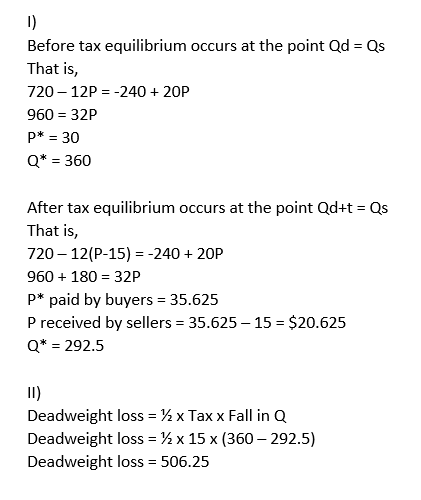##### Add Answer of: 1) Assume that the market demand and supply functions for Nice to See book factory shelves...
Similar Homework Help Questions
• ### 5. Consider a market in which demand and supply have the following functional forms: The free-market...

5. Consider a market in which demand and supply have the following functional forms: The free-market equilibrium is at P = \$24 and Q = 12. Qd = 24-1/2PB and Qs = -12+PS a. Graph the free market equilibrium in the space below. Label the curves and show the values of ALL intercepts (show your work to find them). b. Now suppose that the Government decides to impose a \$6 per-unit subsidy in this market. Calculate the price paid by...

• ### 5. Consider a market in which demand and supply have the following functional forms: The free-market...

5. Consider a market in which demand and supply have the following functional forms: The free-market equilibrium is at P = \$24 and Q = 12. Qd = 24-1/2PB and Qs = -12+PS a. Graph the free market equilibrium in the space below. Label the curves and show the values of ALL intercepts (show your work to find them). b. Now suppose that the Government decides to impose a \$6 per-unit subsidy in this market. Calculate the price paid by...

• ### A market for baby bottles has the following supply and demand functions qS = −6 +...

A market for baby bottles has the following supply and demand functions qS = −6 + 3p qD = 14 − 2p a. Now, suppose a per unit tax of 5 were charged to the buyer. What are the equilibrium quantity, price paid by the buyer, and price received by the seller? b. How much tax revenue is raised? How much of that tax burden is borne by the buyer? c. Calculate the Consumer Surplus, Producer Surplus, Total Welfare Level,...

• ### Suppose that the market for a children’s book is given by the following demand and supply...

Suppose that the market for a children’s book is given by the following demand and supply functions: Demand:          QD= 98 - 4P    Supply:             QS= -4 + 2P            Where: QD and QS are quantity demand and quantity supplied respectively, and P is the price. The equilibrium price is \$______

• ### Suppose that the market for a children’s book is given by the following demand and supply...

Suppose that the market for a children’s book is given by the following demand and supply functions: Demand:         QD= 98 - 4P Supply:             QS= -4 + 2P            Where: QD and QS are quantity demand and quantity supplied respectively, and P is the price. The equilibrium quantity is ______ units

• ### Q3) Suppose that the market demand and supply curve in a competitive market are Q"-15 - 2P and QS...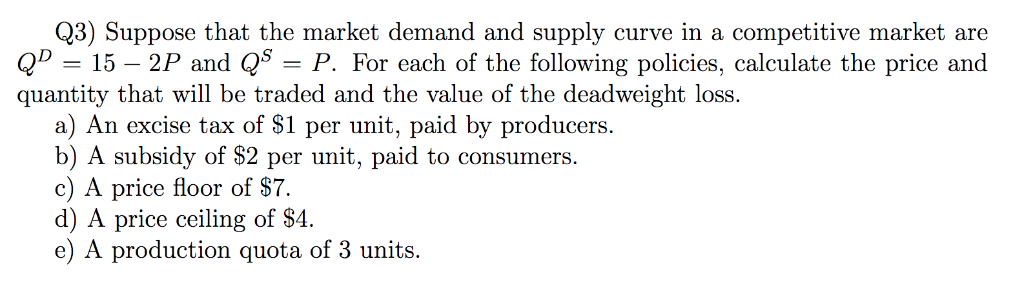Q3) Suppose that the market demand and supply curve in a competitive market are Q"-15 - 2P and QS-P. For each of the following policies, calculate the price and quantity that will be traded and the value of the deadweight loss. a) An excise tax of S1 per unit, paid by producers. b) A subsidy of \$2 per unit, paid to consumers. c) A price floor of S7. d) A price ceiling of S4. e) A production quota of 3...

• ### Question 5 Consider the market for rice, which is perfectly competitive. Aggregate supply and demand are...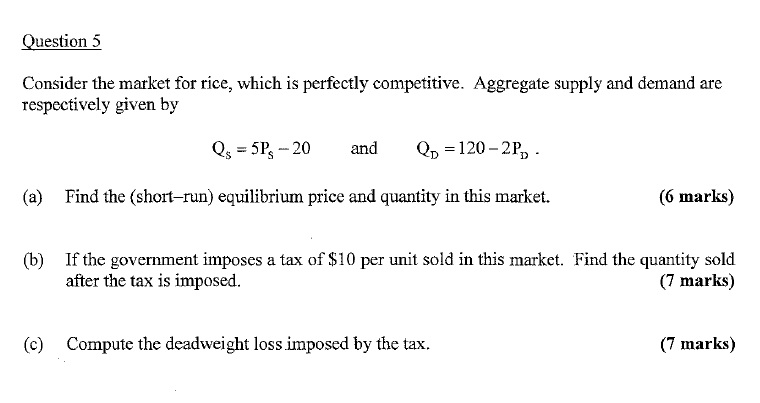Question 5 Consider the market for rice, which is perfectly competitive. Aggregate supply and demand are respectively given by Qs -5P 20 and 120-2P, (a) Find the (short-run) equilibrium price and quantity in this market. (6 marks) If the government imposes a tax of S10 per unit sold in this market. Find the quantity sold after the tax is imposed. (b) (7 marks) (c) Compute the deadweight loss imposed by the tax. (7 marks)

• ### 1. Numerical analysis of supply and demand: Consider the following demand and supply functions that provide...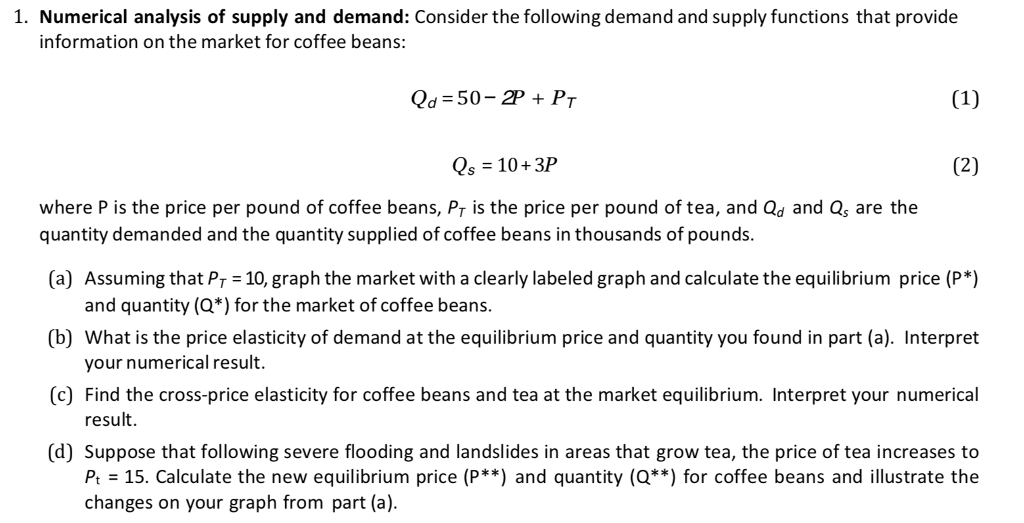1. Numerical analysis of supply and demand: Consider the following demand and supply functions that provide information on the market for coffee beans: Qd 50-2PPr Qs 10+3P where P is the price per pound of coffee beans, Pr is the price per pound of tea, and Qd and Qs are the quantity demanded and the quantity supplied of coffee beans in thousands of pounds. a Assuming that Pr 10, graph the market with a clearly labeled graph and calculate the...

• ### 1. Numerical analysis of supply and demand: Consider the following demand and supply functions that provide...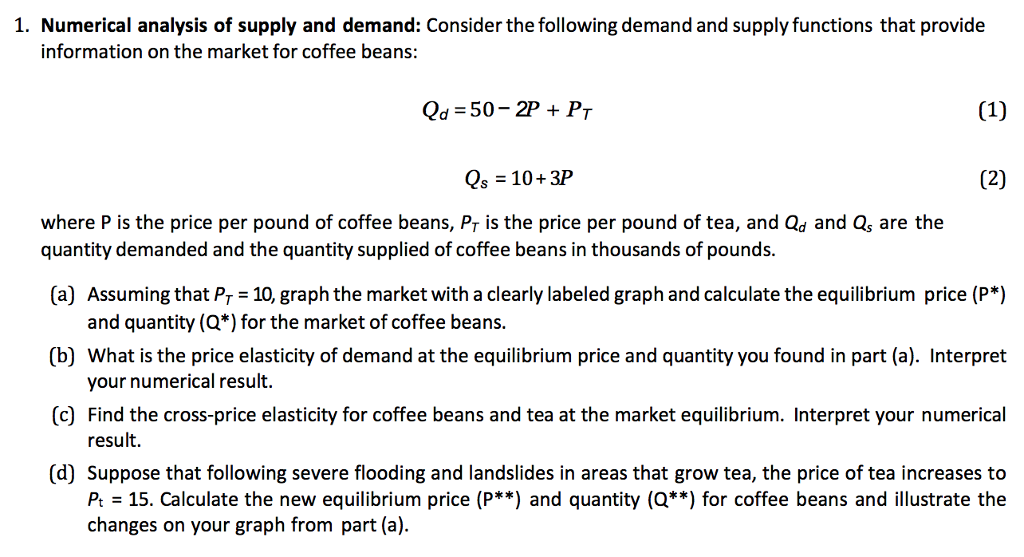1. Numerical analysis of supply and demand: Consider the following demand and supply functions that provide information on the market for coffee beans: Qd 50- 2P PT Qs 10+3P where P is the price per pound of coffee beans, Pr is the price per pound of tea, and Qd and Qs are the quantity demanded and the quantity supplied of coffee beans in thousands of pounds. (a) Assuming that Pr 10, graph the market with a clearly labeled graph and...

• ### The equations represent the demand and supply for silver pendants. The government is considering imposing a...

The equations represent the demand and supply for silver pendants. The government is considering imposing a \$4 per unit tax of buyers of silver pendants. QD= 50,000-2,000P QS= -10,000+2,000P QD (with tax)= 50,000-2,000(P+T) 1. What is the before tax equilibrium price (P) and quantity (Q- in thousands) of pendants? a. P= \$50; Q= 10 thousand b. P= \$20; Q= 15 thousand c. P= \$15; Q= 20 thousand d. P= \$10; Q= 30 thousand. 2. Who carries the highest incidence of...

Need Online Homework Help?